Скачать презентацию Constraint operations Simplification Optimization and Implication 1

ad72cb32df670bb1888c9b1b0c3ef61d.ppt

• Количество слайдов: 36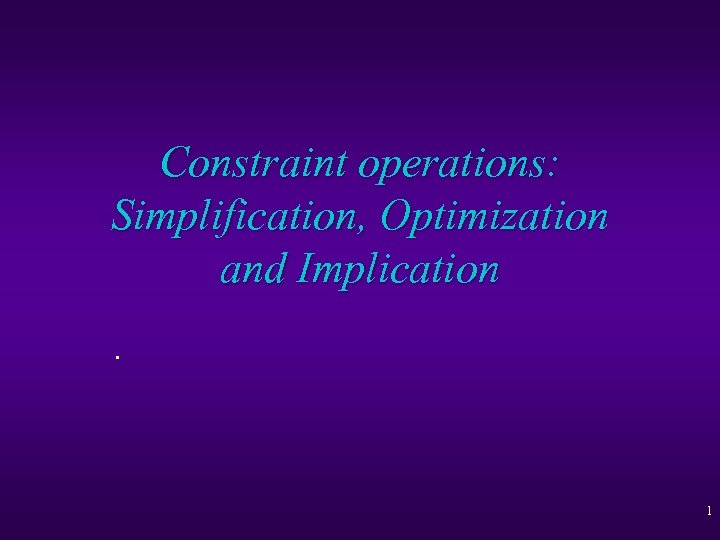Constraint operations: Simplification, Optimization and Implication. 1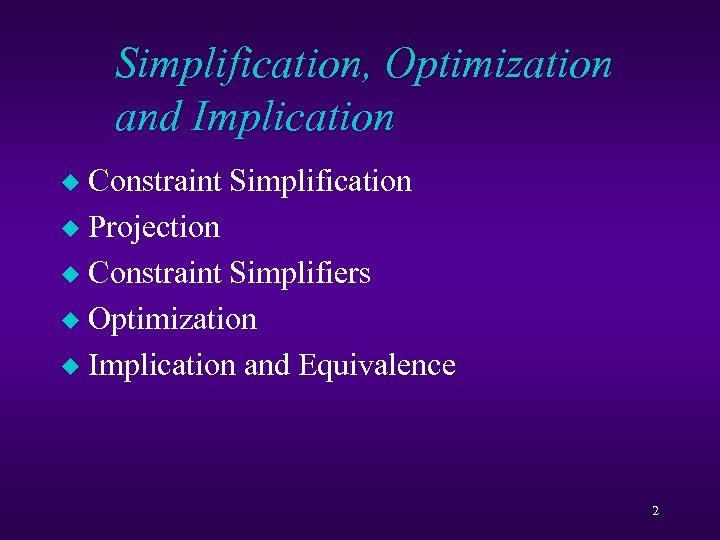Simplification, Optimization and Implication Constraint Simplification u Projection u Constraint Simplifiers u Optimization u Implication and Equivalence u 2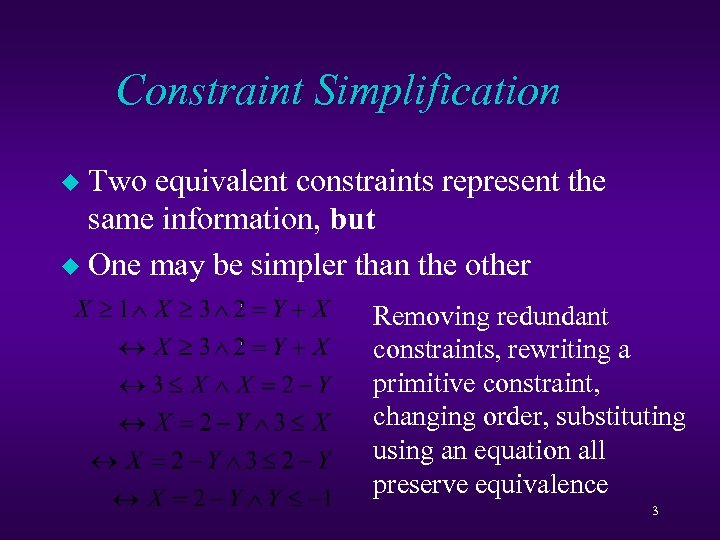Constraint Simplification Two equivalent constraints represent the same information, but u One may be simpler than the other u Removing redundant constraints, rewriting a primitive constraint, changing order, substituting using an equation all preserve equivalence 3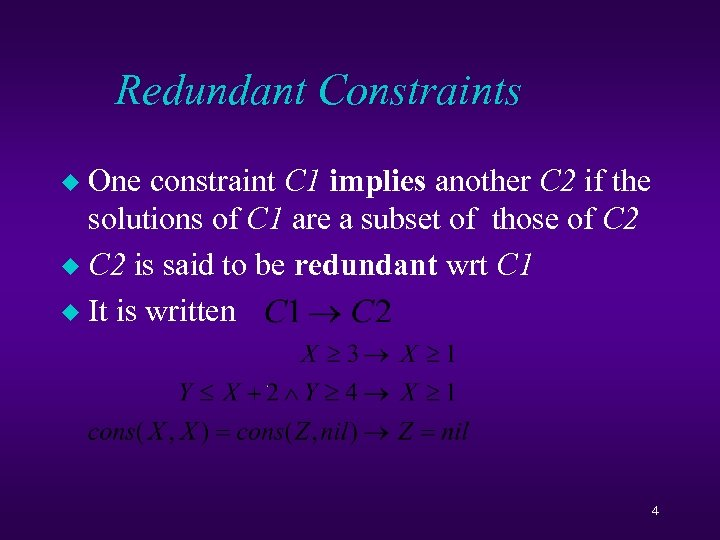Redundant Constraints One constraint C 1 implies another C 2 if the solutions of C 1 are a subset of those of C 2 u C 2 is said to be redundant wrt C 1 u It is written u 4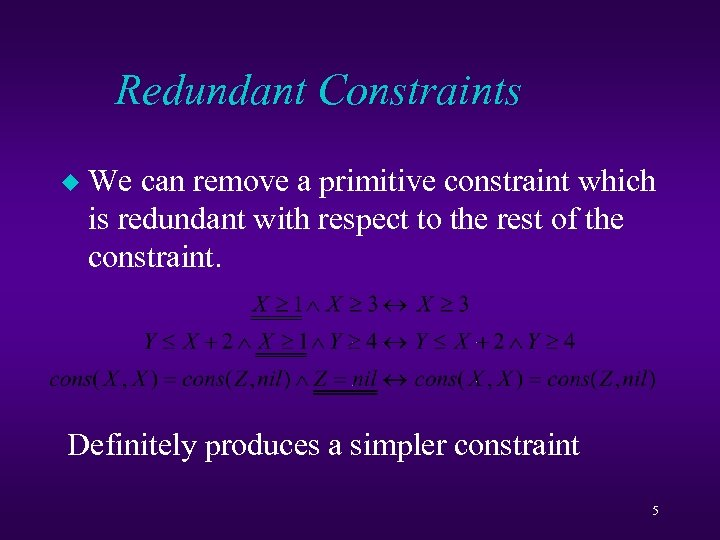Redundant Constraints u We can remove a primitive constraint which is redundant with respect to the rest of the constraint. Definitely produces a simpler constraint 5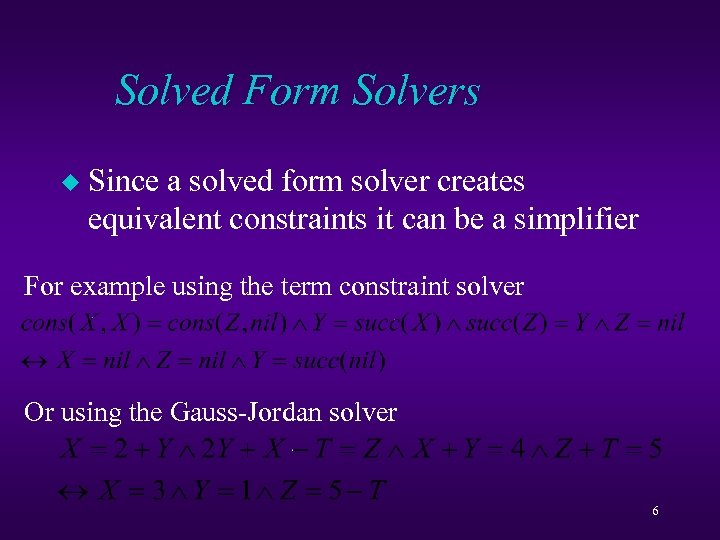Solved Form Solvers u Since a solved form solver creates equivalent constraints it can be a simplifier For example using the term constraint solver Or using the Gauss-Jordan solver 6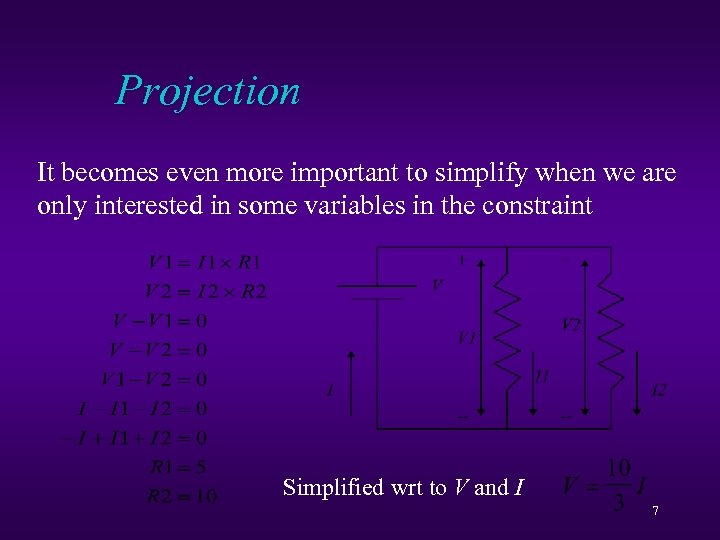Projection It becomes even more important to simplify when we are only interested in some variables in the constraint Simplified wrt to V and I 7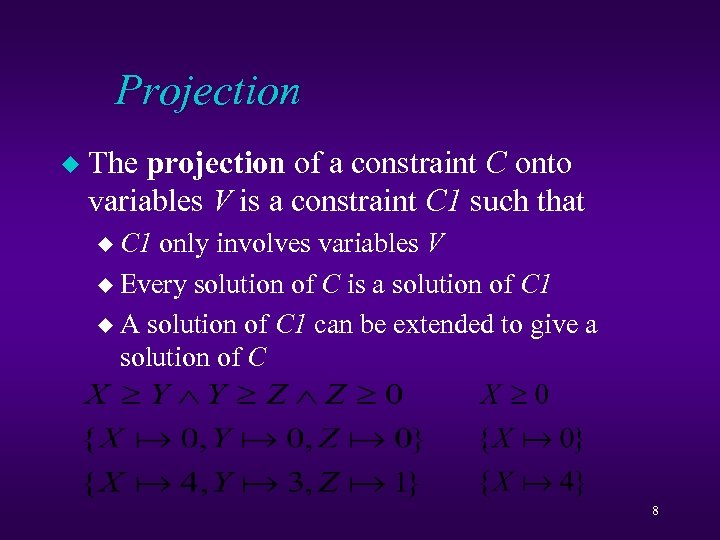Projection u The projection of a constraint C onto variables V is a constraint C 1 such that u C 1 only involves variables V u Every solution of C is a solution of C 1 u A solution of C 1 can be extended to give a solution of C 8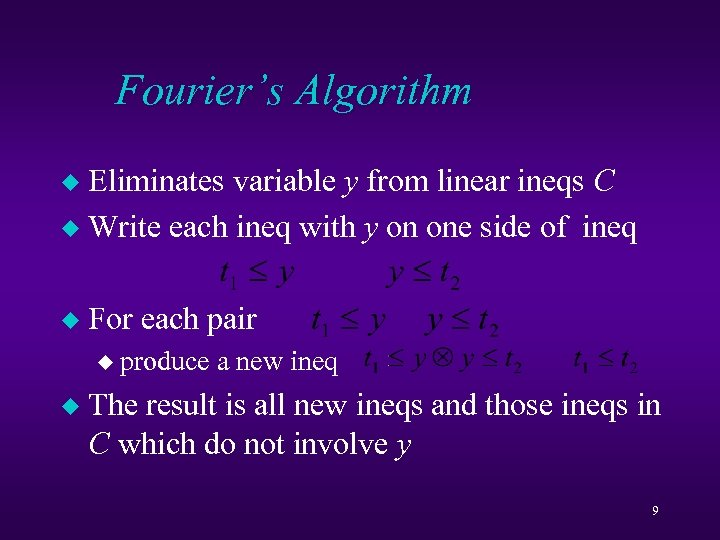Fourier’s Algorithm Eliminates variable y from linear ineqs C u Write each ineq with y on one side of ineq u u For each pair u produce u a new ineq The result is all new ineqs and those ineqs in C which do not involve y 9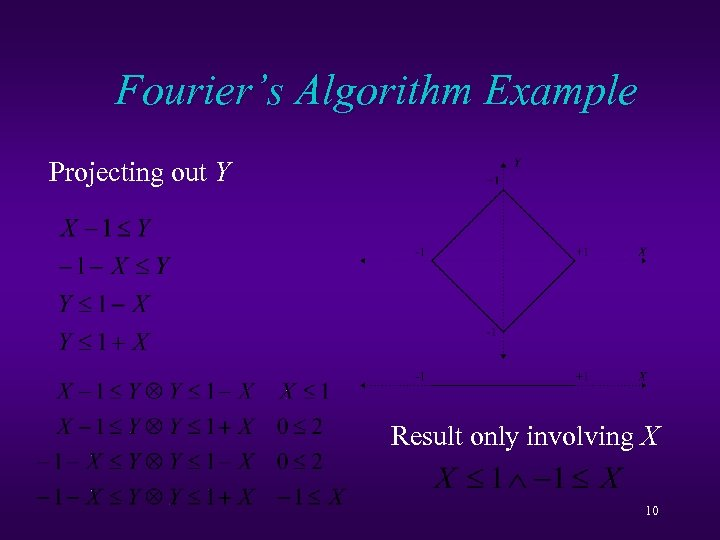Fourier’s Algorithm Example Projecting out Y Result only involving X 10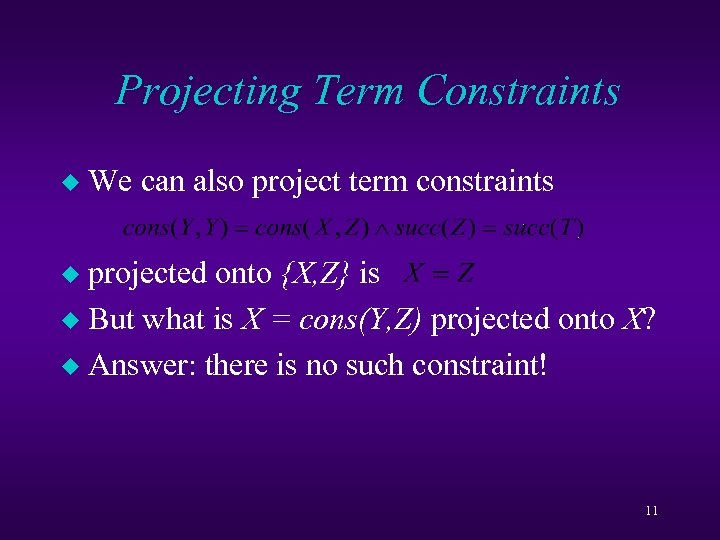Projecting Term Constraints u We can also project term constraints projected onto {X, Z} is u But what is X = cons(Y, Z) projected onto X? u Answer: there is no such constraint! u 11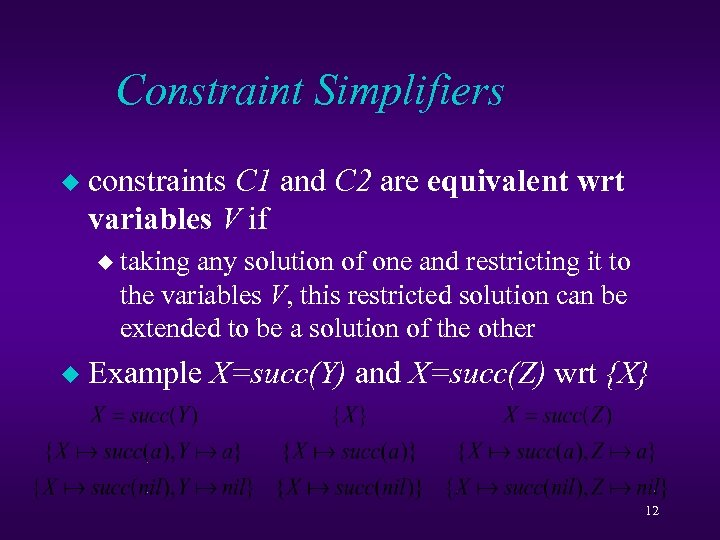Constraint Simplifiers u constraints C 1 and C 2 are equivalent wrt variables V if u taking any solution of one and restricting it to the variables V, this restricted solution can be extended to be a solution of the other u Example X=succ(Y) and X=succ(Z) wrt {X} 12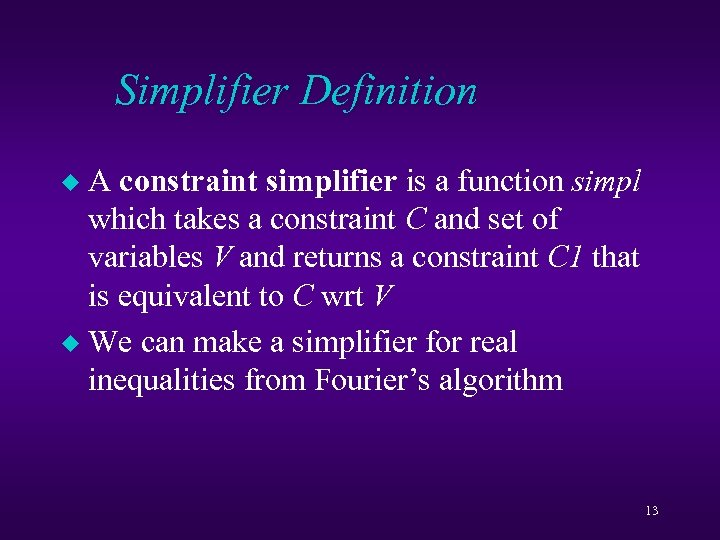Simplifier Definition A constraint simplifier is a function simpl which takes a constraint C and set of variables V and returns a constraint C 1 that is equivalent to C wrt V u We can make a simplifier for real inequalities from Fourier’s algorithm u 13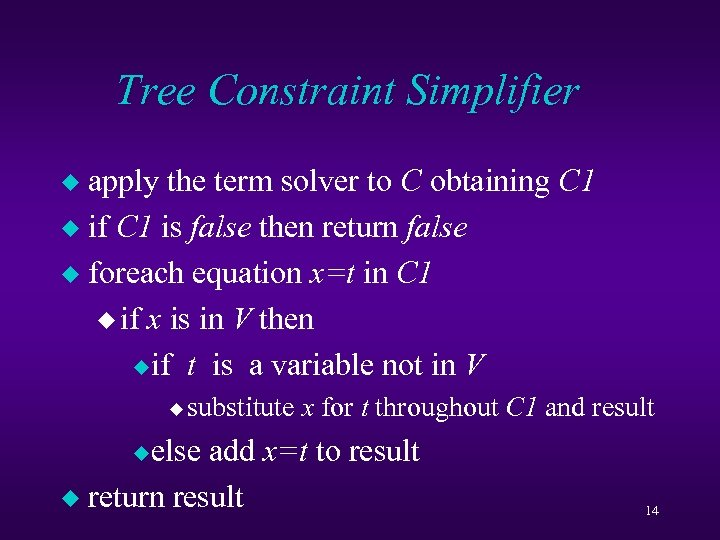Tree Constraint Simplifier apply the term solver to C obtaining C 1 u if C 1 is false then return false u foreach equation x=t in C 1 u if x is in V then u if t is a variable not in V u u substitute x for t throughout C 1 and result u else add x=t to result u return result 14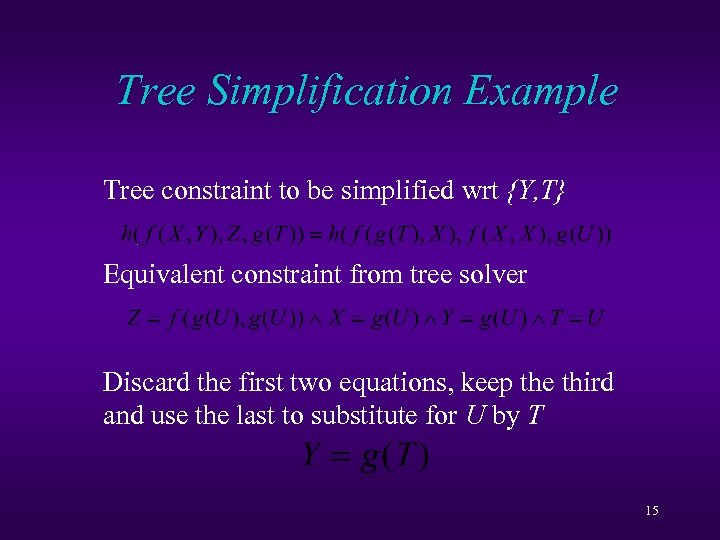Tree Simplification Example Tree constraint to be simplified wrt {Y, T} Equivalent constraint from tree solver Discard the first two equations, keep the third and use the last to substitute for U by T 15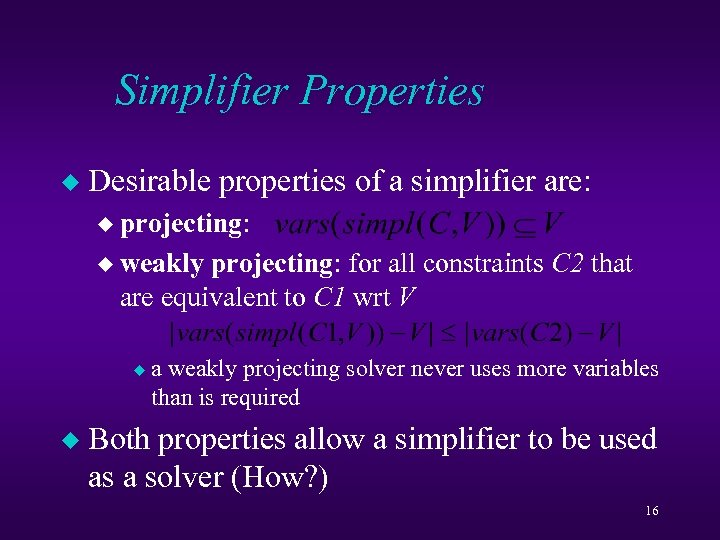Simplifier Properties u Desirable properties of a simplifier are: u projecting: u weakly projecting: for all constraints C 2 that are equivalent to C 1 wrt V u u a weakly projecting solver never uses more variables than is required Both properties allow a simplifier to be used as a solver (How? ) 16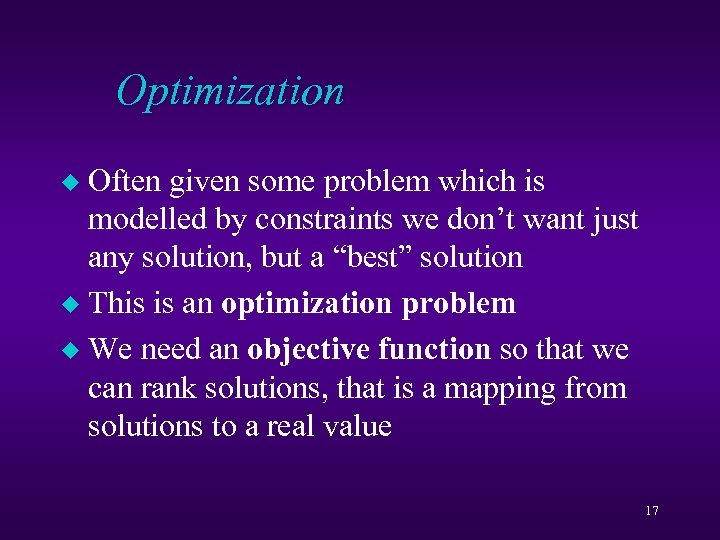Optimization Often given some problem which is modelled by constraints we don’t want just any solution, but a “best” solution u This is an optimization problem u We need an objective function so that we can rank solutions, that is a mapping from solutions to a real value u 17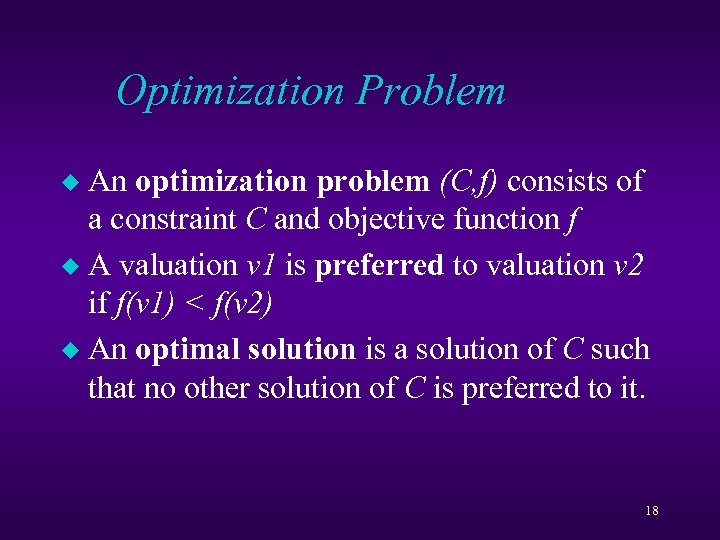Optimization Problem An optimization problem (C, f) consists of a constraint C and objective function f u A valuation v 1 is preferred to valuation v 2 if f(v 1) < f(v 2) u An optimal solution is a solution of C such that no other solution of C is preferred to it. u 18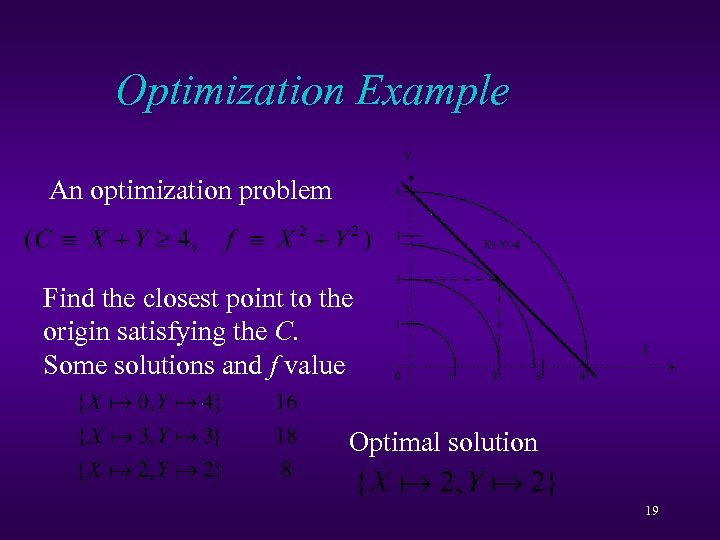Optimization Example An optimization problem Find the closest point to the origin satisfying the C. Some solutions and f value Optimal solution 19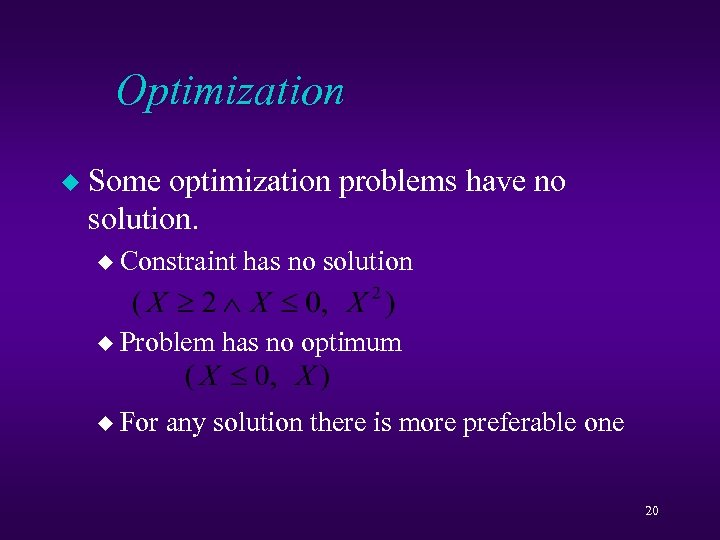Optimization u Some optimization problems have no solution. u Constraint u Problem u For has no solution has no optimum any solution there is more preferable one 20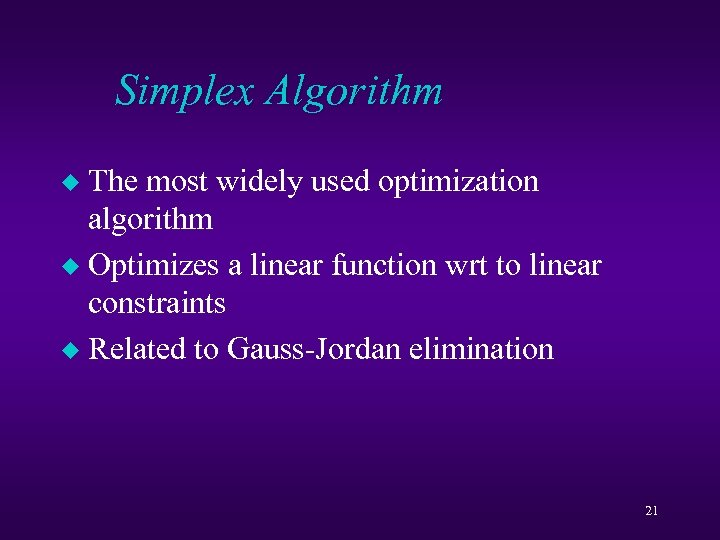Simplex Algorithm The most widely used optimization algorithm u Optimizes a linear function wrt to linear constraints u Related to Gauss-Jordan elimination u 21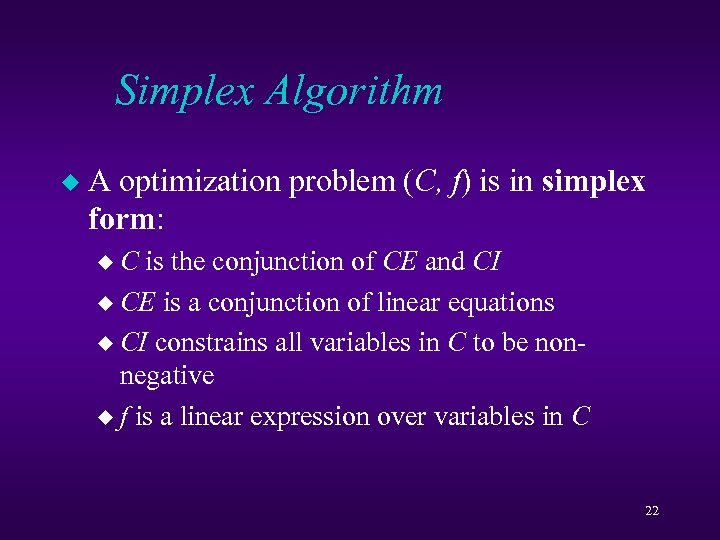Simplex Algorithm u A optimization problem (C, f) is in simplex form: u. C is the conjunction of CE and CI u CE is a conjunction of linear equations u CI constrains all variables in C to be nonnegative u f is a linear expression over variables in C 22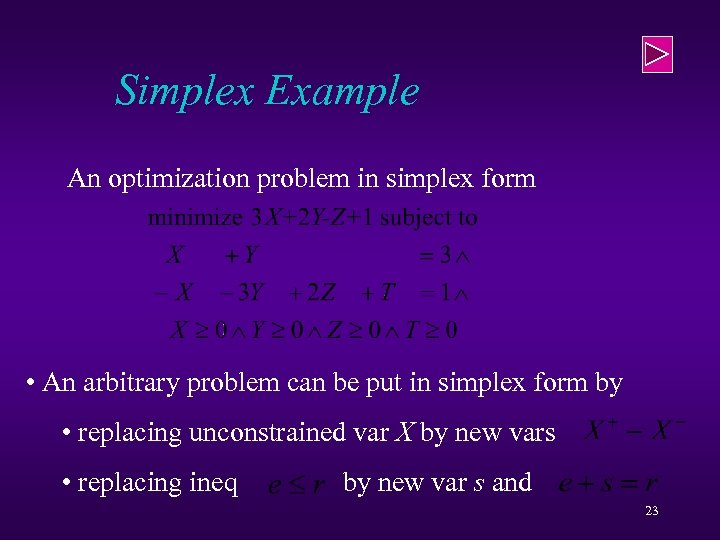Simplex Example An optimization problem in simplex form • An arbitrary problem can be put in simplex form by • replacing unconstrained var X by new vars • replacing ineq by new var s and 23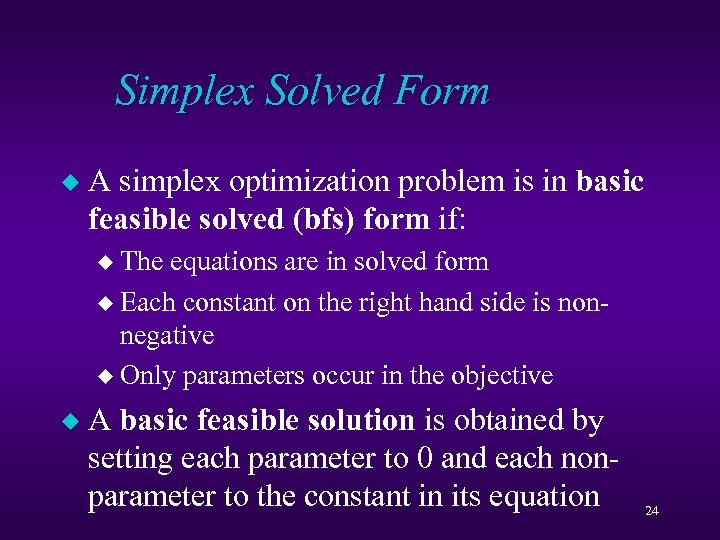Simplex Solved Form u A simplex optimization problem is in basic feasible solved (bfs) form if: u The equations are in solved form u Each constant on the right hand side is nonnegative u Only parameters occur in the objective u A basic feasible solution is obtained by setting each parameter to 0 and each nonparameter to the constant in its equation 24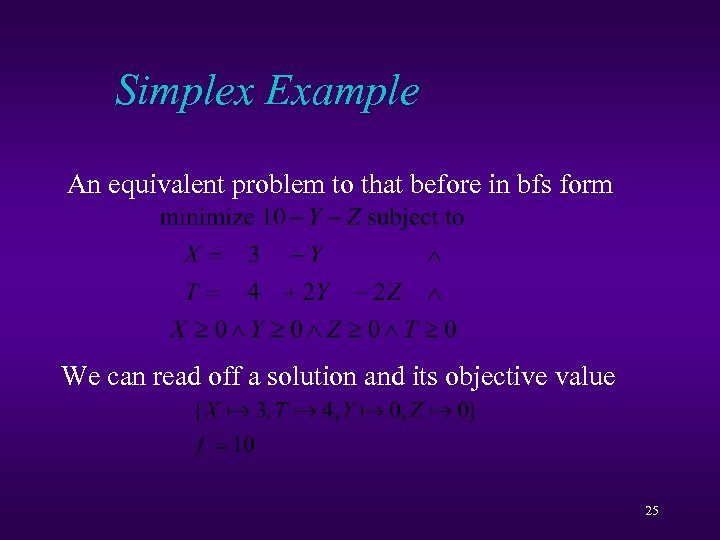Simplex Example An equivalent problem to that before in bfs form We can read off a solution and its objective value 25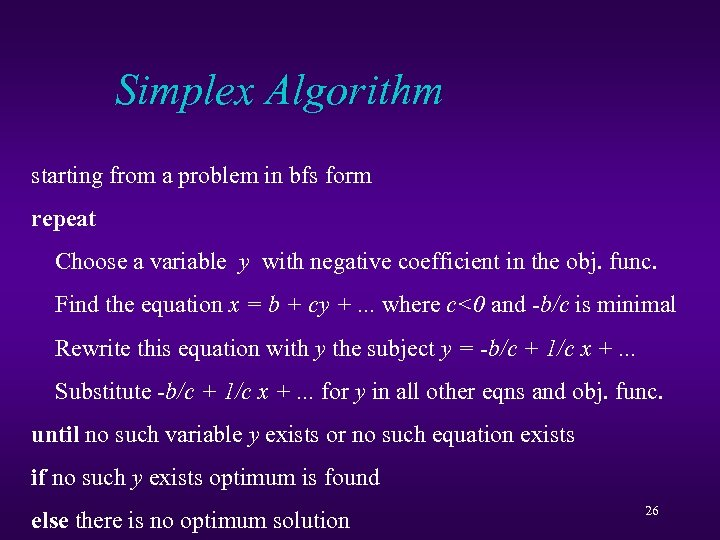Simplex Algorithm starting from a problem in bfs form repeat Choose a variable y with negative coefficient in the obj. func. Find the equation x = b + cy +. . . where c<0 and -b/c is minimal Rewrite this equation with y the subject y = -b/c + 1/c x +. . . Substitute -b/c + 1/c x +. . . for y in all other eqns and obj. func. until no such variable y exists or no such equation exists if no such y exists optimum is found else there is no optimum solution 26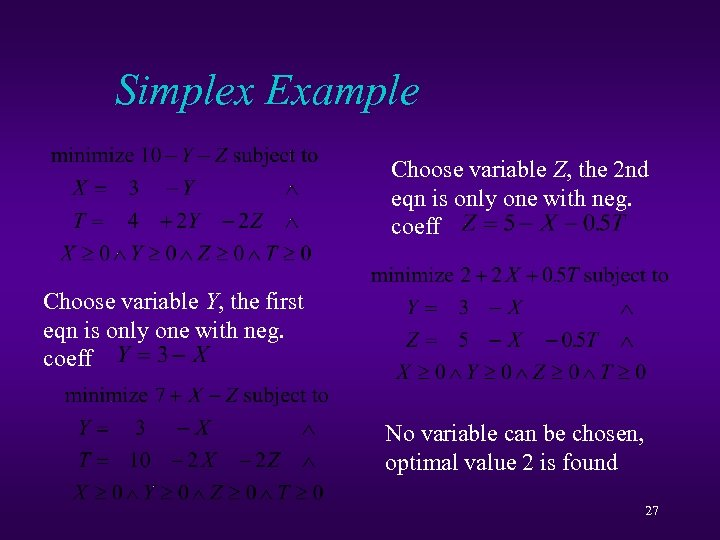Simplex Example Choose variable Z, the 2 nd eqn is only one with neg. coeff Choose variable Y, the first eqn is only one with neg. coeff No variable can be chosen, optimal value 2 is found 27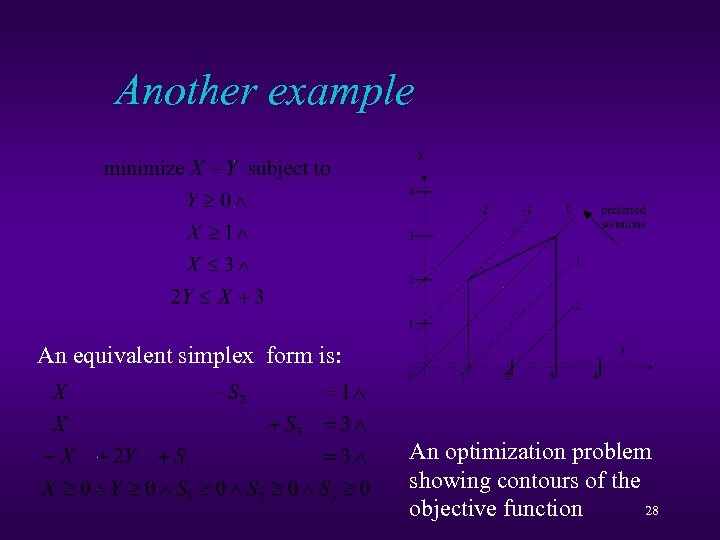Another example An equivalent simplex form is: An optimization problem showing contours of the 28 objective function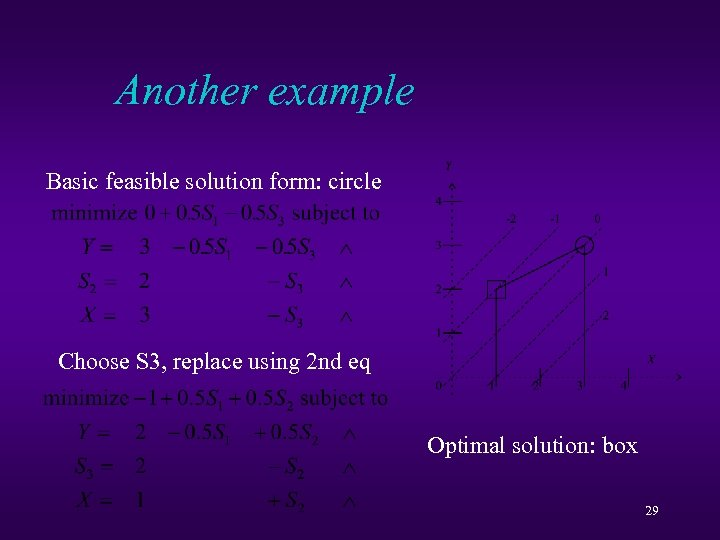Another example Basic feasible solution form: circle Choose S 3, replace using 2 nd eq Optimal solution: box 29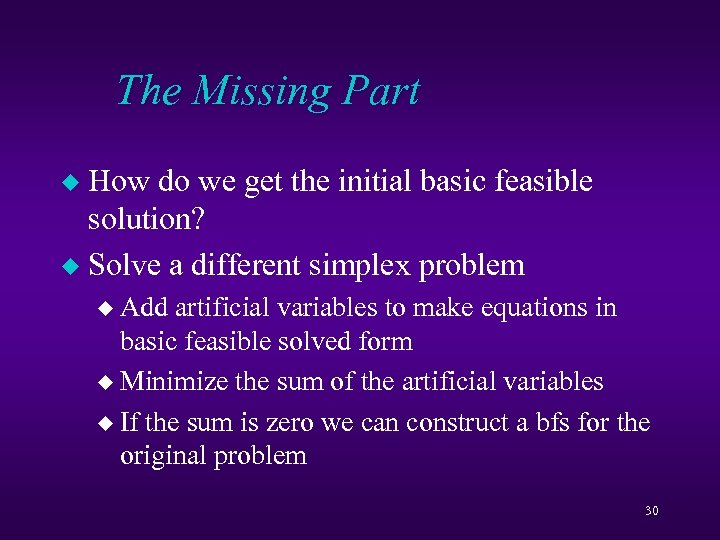The Missing Part How do we get the initial basic feasible solution? u Solve a different simplex problem u u Add artificial variables to make equations in basic feasible solved form u Minimize the sum of the artificial variables u If the sum is zero we can construct a bfs for the original problem 30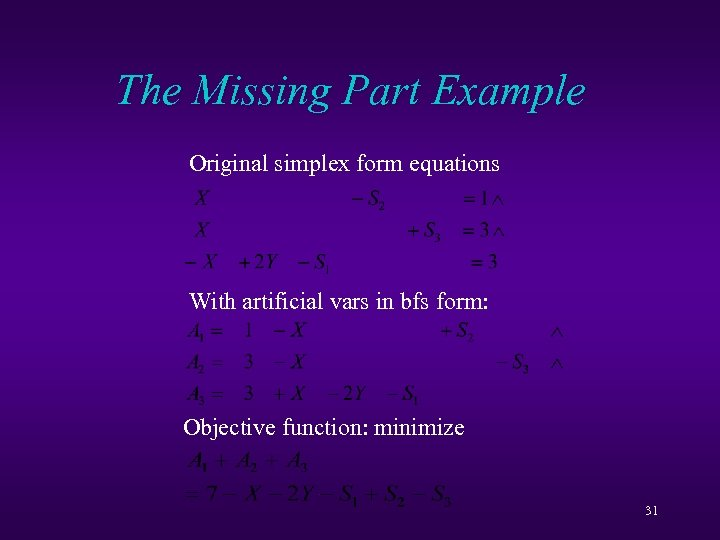The Missing Part Example Original simplex form equations With artificial vars in bfs form: Objective function: minimize 31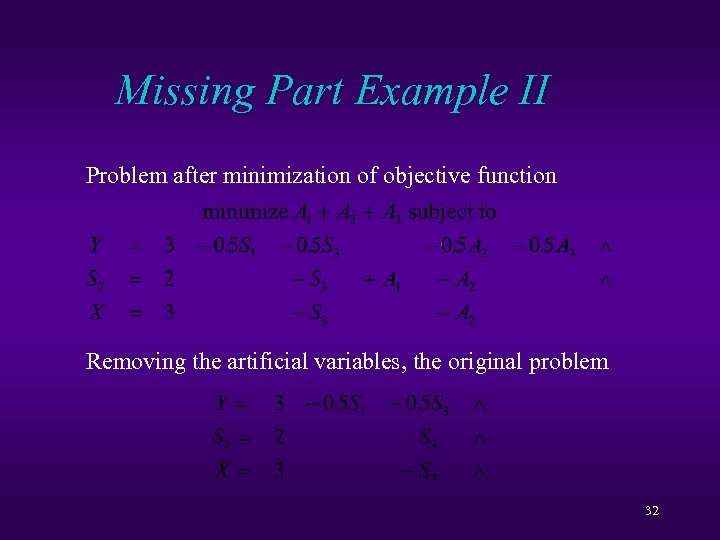Missing Part Example II Problem after minimization of objective function Removing the artificial variables, the original problem 32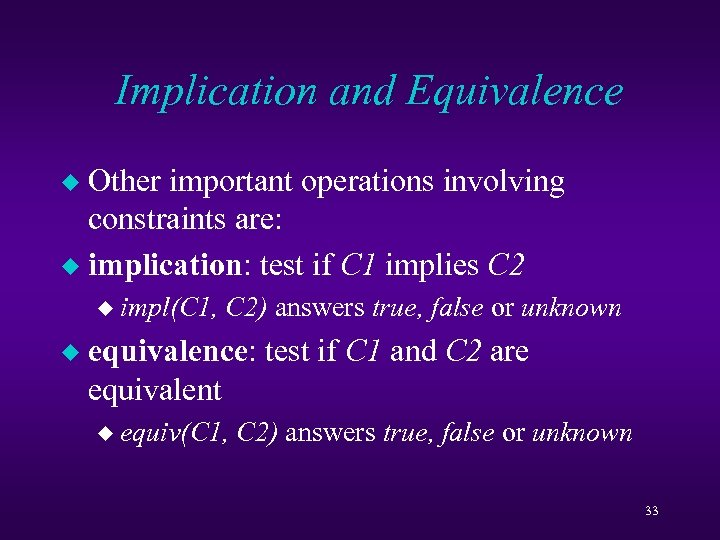Implication and Equivalence Other important operations involving constraints are: u implication: test if C 1 implies C 2 u u impl(C 1, u C 2) answers true, false or unknown equivalence: test if C 1 and C 2 are equivalent u equiv(C 1, C 2) answers true, false or unknown 33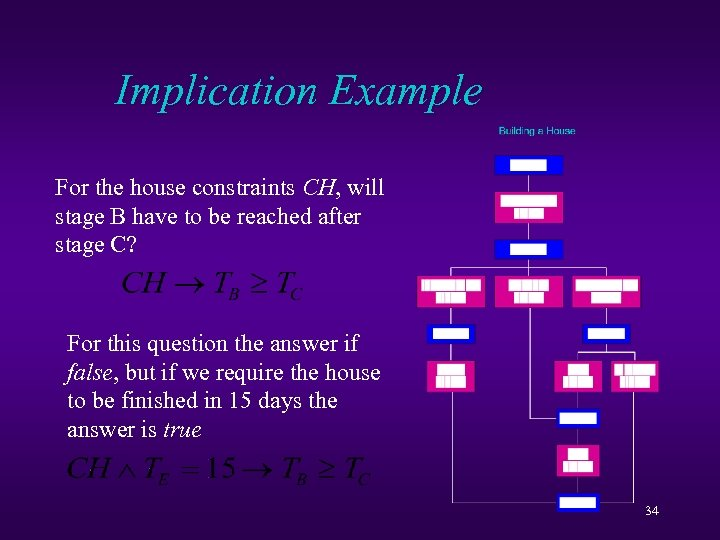Implication Example For the house constraints CH, will stage B have to be reached after stage C? For this question the answer if false, but if we require the house to be finished in 15 days the answer is true 34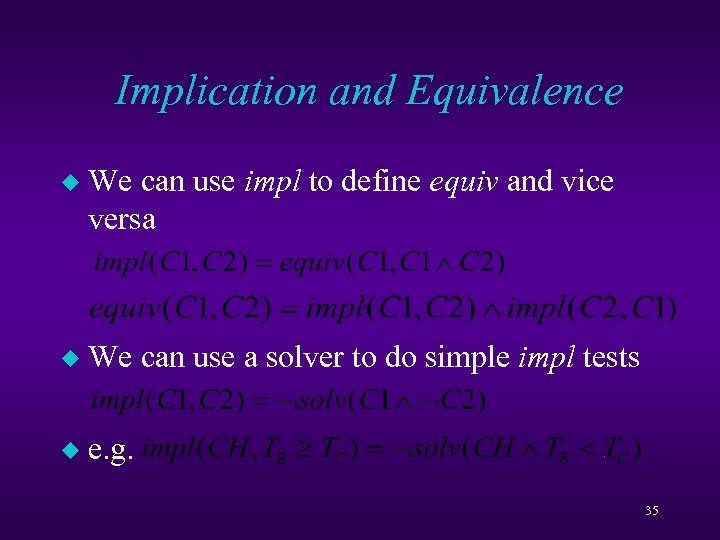Implication and Equivalence u We can use impl to define equiv and vice versa u We can use a solver to do simple impl tests u e. g. 35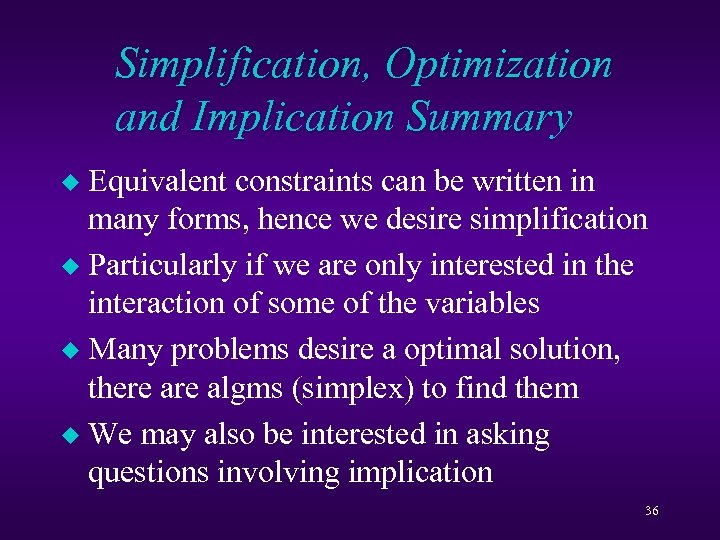Simplification, Optimization and Implication Summary Equivalent constraints can be written in many forms, hence we desire simplification u Particularly if we are only interested in the interaction of some of the variables u Many problems desire a optimal solution, there algms (simplex) to find them u We may also be interested in asking questions involving implication u 36